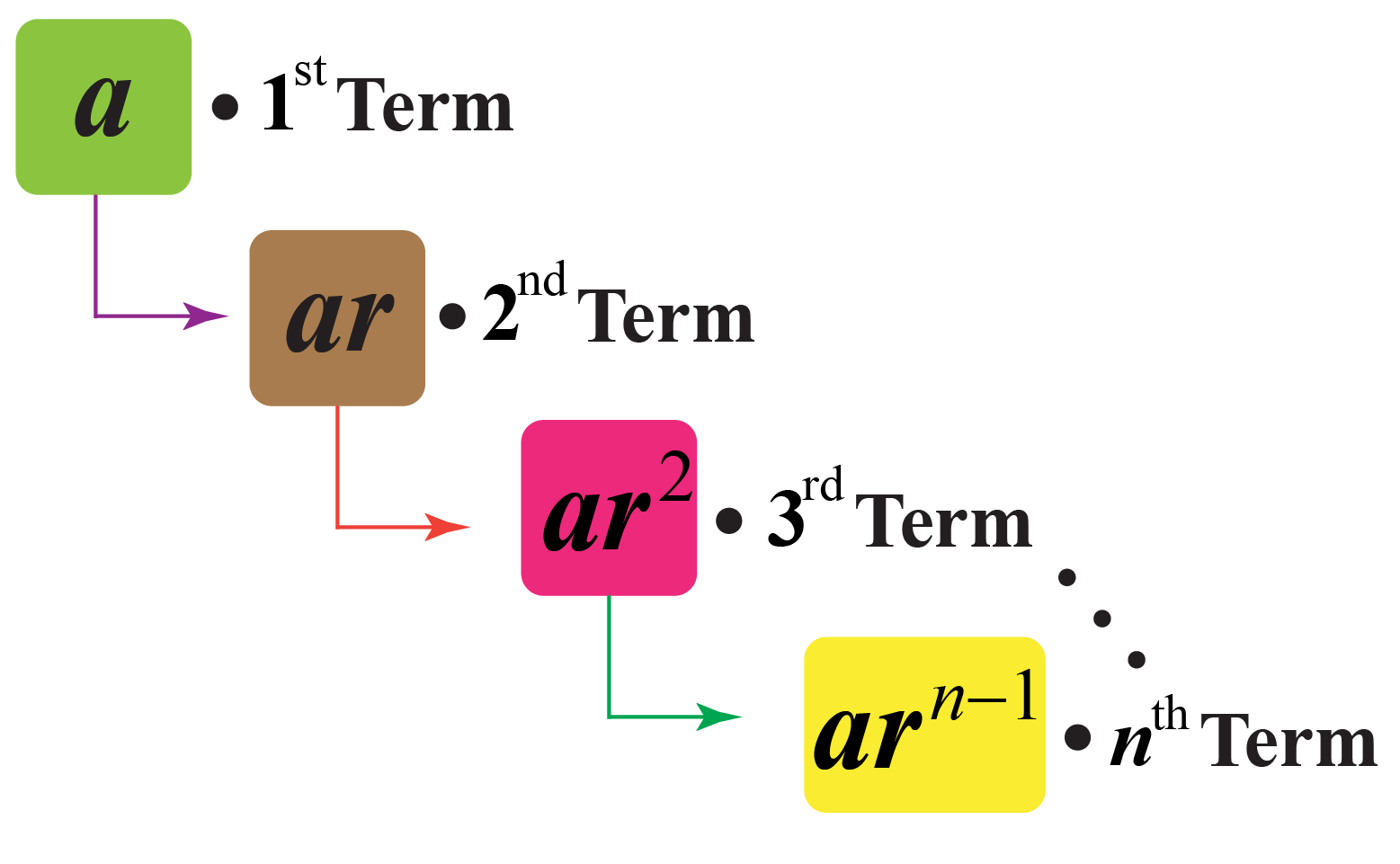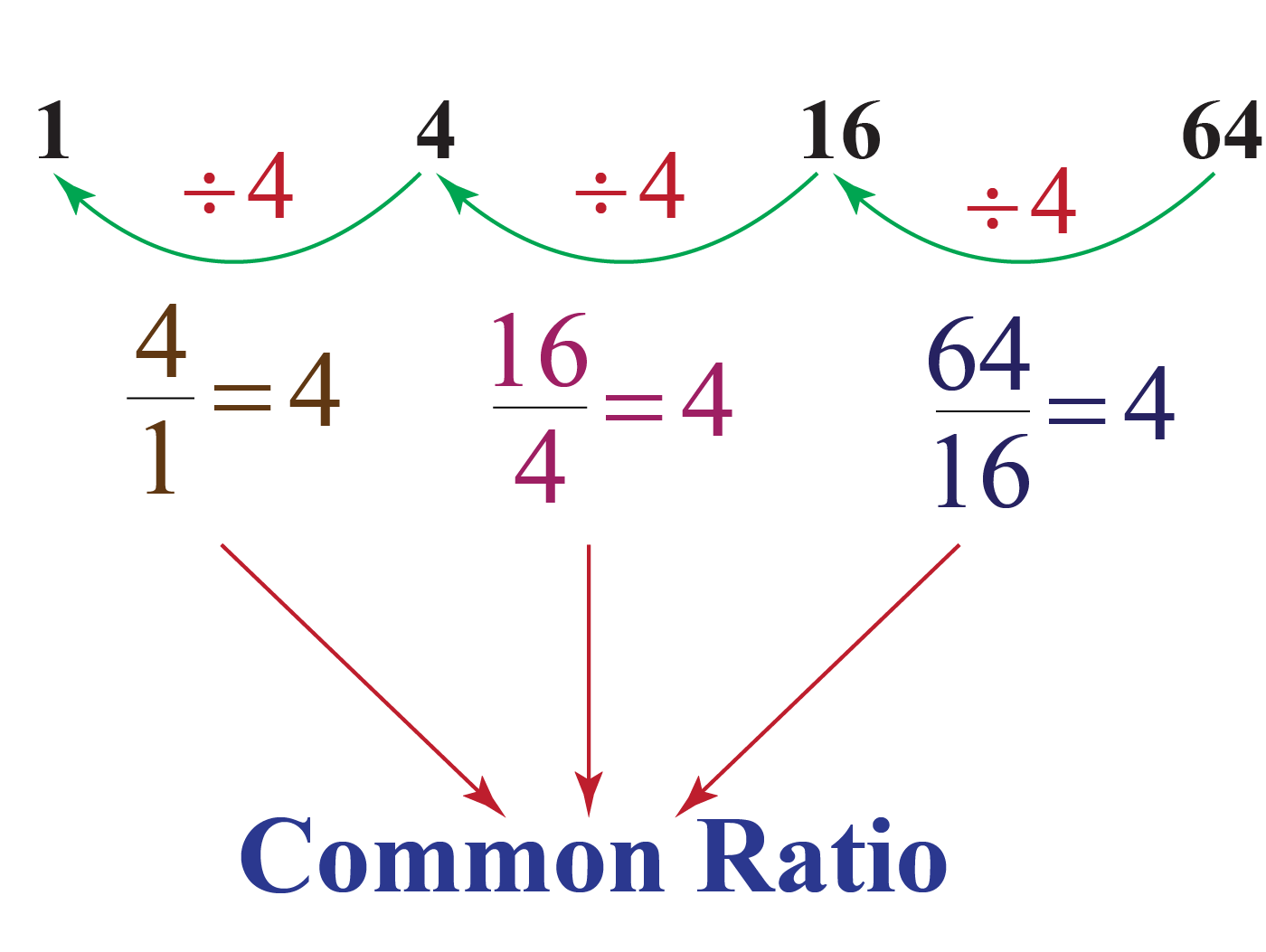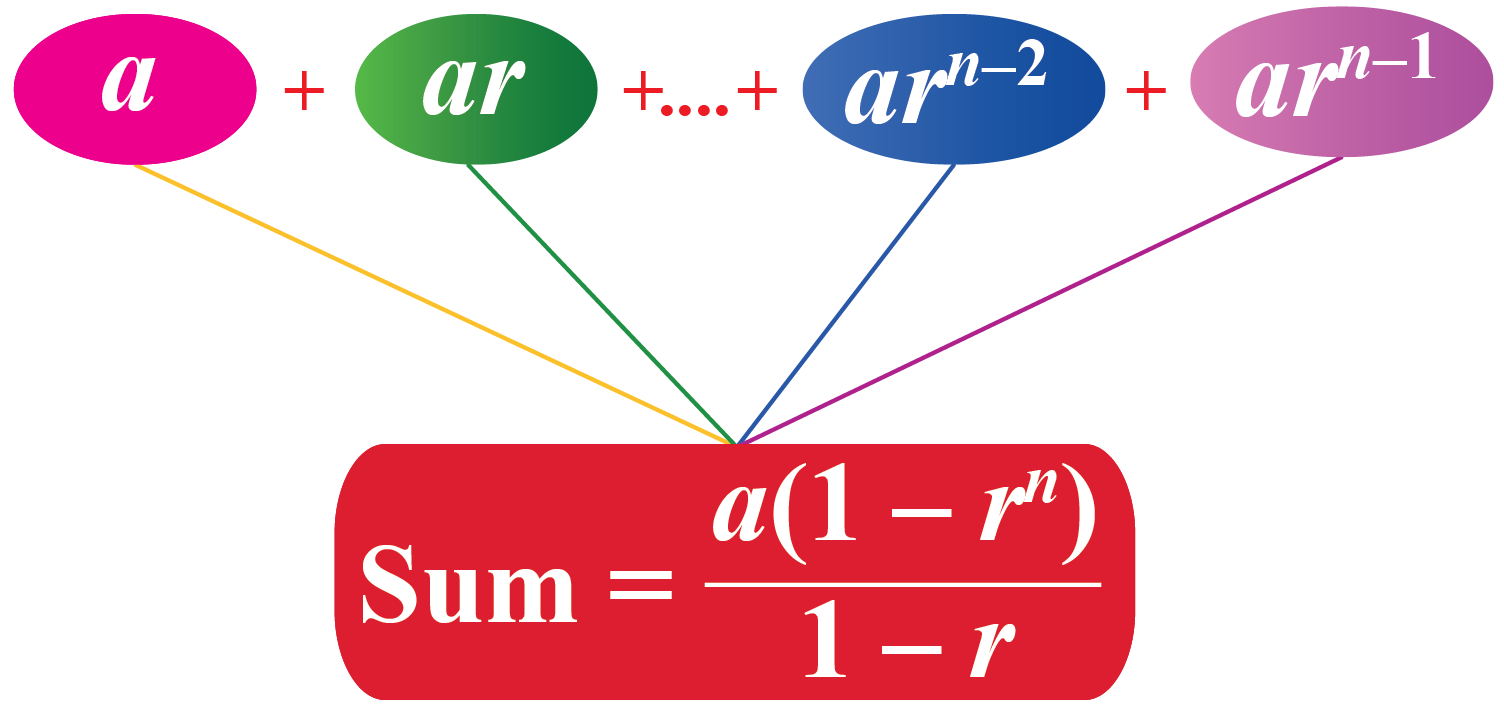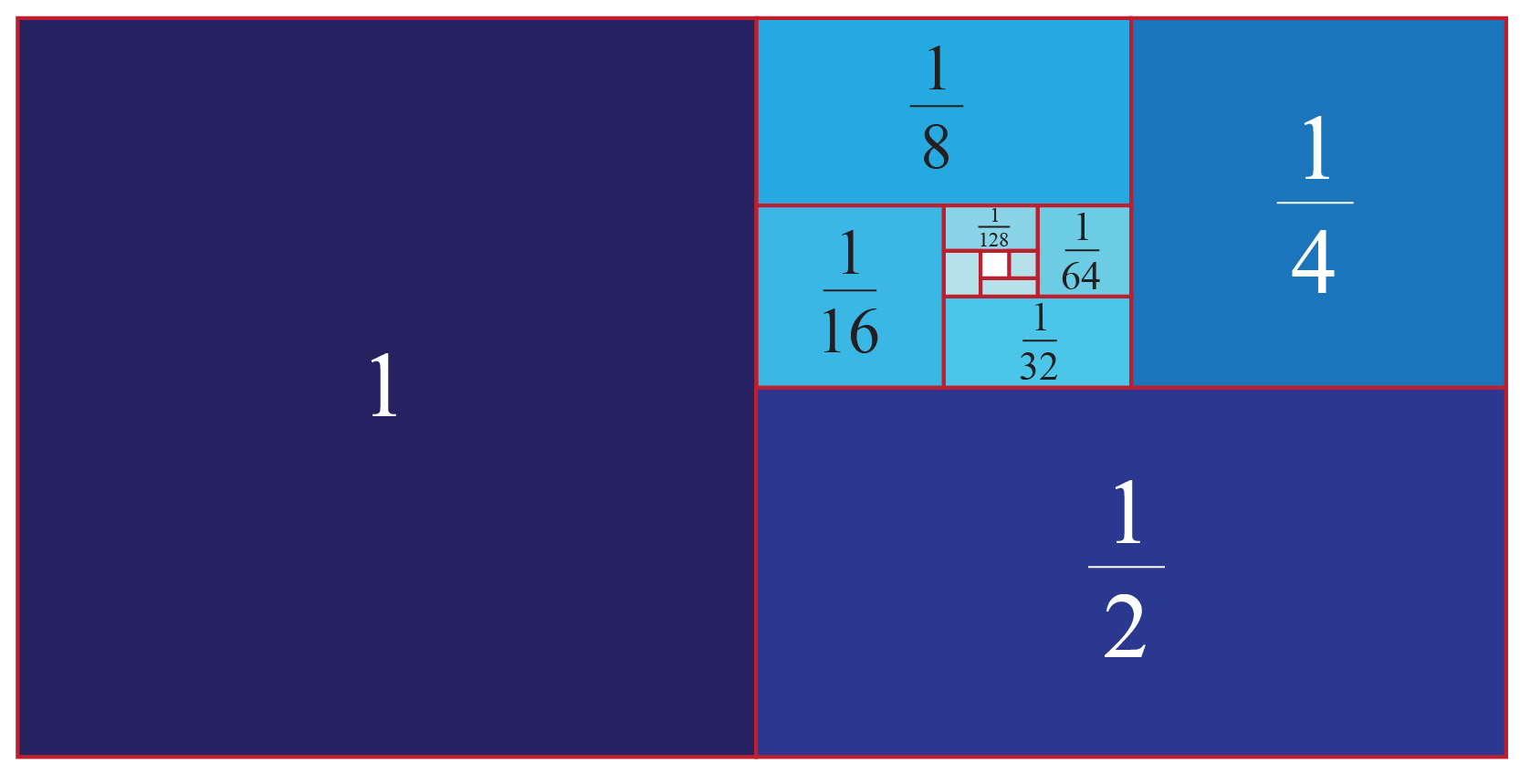# What are Geometric Progressions

John is a brilliant basketball player.

John observes that each time he bounces the ball, the maximum height the ball reaches is reduced by half.He says that if the height attained by the ball in the first bounce is 12 feet, the next time its bounce height would be 6 feet.

Can you determine the height of the ball in its 6th bounce?

To answer this, you need to use the concept of geometric progression.

In this short lesson, we will learn about a special type of sequence, that is, geometric progression.

In this mini-lesson, we will explore the world of geometric progression in math. You will get to learn what is a geometric progression, the sum of infinite terms of GP, the sum of infinite GP formula, the sum of finite GP, geometric progression in real life, geometric progression examples, and other interesting facts around the topic.

## Lesson Plan

 1 What Is Meant by Geometric Progression? 2 Important Notes on Geometric Progression 3 Solved Examples on Geometric Progression 4 Thinking out of the Box! 5 Interactive Questions on Geometric Progression

## What Is Geometric Progression?

A geometric progression is a sequence where every term bears a constant ratio to its preceding term.### Example

Consider an example of geometric progression: 1, 4, 16, 64, ...

Observe that $$\dfrac{4}{1}=\dfrac{16}{4}=\dfrac{64}{16}=4$$Here, 4 is the common ratio of GP.

## What Is the Value of the First term and Common Ratio of GP?

The first term and the common ratio of a GP are denoted as $$a$$ and $$r$$ respectively.

The nth term of a GP is given by the formula:

 $$a_n=ar^{n-1}$$

The first term of the GP is calculated by substituting $$n=1$$ in the formula $$a_n=ar^{n-1}$$.

The common ratio is calculated by finding the ratio of any term by its preceding term.

For example, consider the G.P. $$2, 4, 8, ...$$

The first term of the GP is 2 and the common ratio is $$\dfrac{4}{2}=2$$

Use the simulation below to calculate the nth term of some geometric progressions.

Input the values of $$n$$ for the number of terms you want to calculate.

## How To Calculate the Sum of Finite GP?

Let's say we have a G.P. with the first term $$a$$ and the common ratio $$r$$ and it has a finite number of terms.

Let $$n$$ terms of the G.P. be $$a, ar, ar^2, ... , ar^{n-1}$$

So, the sum of this G.P. is $$S_{n}=a+ar+ar^2+ ... +ar^{n-1}\;\;\;\;\cdots(1)$$

If $$r=1$$, then $$S_{n}=a+a+a+ ... +a(n \text{ times})=na$$

If $$r \neq 1$$, multiply the equation (1) by $$r$$.

Then, $$rS_{n}=ar+ar^2+ar^3+ ... +ar^{n}\;\;\;\;\cdots(2)$$

Subtract equation (2) from equation (1).

\begin{align}S_{n}-rS_{n}&=a+ar+ar^2+ ... +ar^{n-1}-(ar+ar^2+ar^3+ ... +ar^{n})\\(1-r)S_{n}&=a-ar^{n}\\S_n&=\dfrac{a(1-r^{n})}{1-r}\end{align}If $$r=1$$, $$S_{n}=na$$ If $$r \neq 1$$, $$S_{n}=\dfrac{a(1-r^{n})}{1-r}$$

## How to Determine the Sum of Infinite GP?

Case 1: Common ratio is less than 1 (r <1)

Let's say we have a G.P. with first term $$a$$ and common ratio $$r$$ and it has an infinite number of terms.

$$a, ar, ar^2, ...$$

So, the sum of this G.P. is $$S_{n}=a+ar+ar^2+ ...$$

The sum of infinite terms of G.P. $$S_n$$ is calculated by using the formula:

 $$S_n=\dfrac{a}{1-r}$$

Case 2: Common ratio is greater than 1 (r >1)

Let's say we have a G.P. with first term $$a$$ and common ratio $$r$$ and it has an infinite number of terms.

$$a, ar, ar^2, ...$$

So, the sum of this G.P. is $$S_{n}=a+ar+ar^2+ ...$$

Now since $$r>1$$, the geometric series is divergent and its successive terms keep on increasing.

Therefore the sum of the GP can not be calculated.

## Geometric Progression in Real Life

Some real-life examples of GP are as follows:

• The population growth rate when each couple decides to have only 1 kid.
• Each half-life of a radioactive substance, compound interest on the amount deposited in a bank.
• Height of a ball bouncing at a fixed percentage of its previous height after it hits the ground each time.

## Solved Examples

 Example 1

Look at the pattern shown below.Observe that each square is half of the size of the square next to it.

Which sequence does this pattern represent?

### Solution

Let's write the sequence represented in the figure.

$1, \dfrac{1}{2}, \dfrac{1}{4}, \dfrac{1}{8}, \dfrac{1}{16},...$

Every successive term is obtained by dividing its preceding term by 2

The sequence exhibits a common ratio of $$\dfrac{1}{2}$$

 $$\therefore$$ The pattern represents the geometric progression.
 Example 2

In a certain culture, the count of bacteria gets doubled after every hour.There were 3 bacteria in the culture initially.

What would be the total count of bacteria at the end of the 6th hour?

Solution

Here, the number of bacteria forms a geometric progression where the first term $$a$$ is 3 and the common ratio $$r$$ is 2.

So, the total number of bacteria at the end of the 6th hour will be the sum of the first 6 terms of this progression given by $$S_6$$.

\begin{align}S_6&=\frac{3(2^6-1)}{2-1}\\&=\frac{3(64-1)}{1}\\&=3\times63\\&=189\end{align}

 So, the total count of bacteria at the end of the 6th hour will be 189.
 Example 3

Find the following sums:

(a) $$S = \frac{1}{3} + \frac{1}{9} + \frac{1}{{27}} + ...\,\,{\rm{to }}\;\infty$$

(b) $$S = 1 - \frac{1}{2} + \frac{1}{4} - \frac{1}{8} + ...\,\,\,{\rm{to }}\;\infty$$

Solution

(a) We have:

$a = \frac{1}{3},\,\,\,r = \frac{1}{3}$

Thus,

$S = \frac{a}{{1 - r}} = \frac{{\frac{1}{3}}}{{1 - \frac{1}{3}}} = \frac{1}{2}$

(b) In this case, we have:

$a = 1,\,\,\,r = - \frac{1}{2}$

Thus,

$S = \frac{a}{{1 - r}} = \frac{1}{{1 - \left( { - \frac{1}{2}} \right)}} = \frac{2}{3}$

 $$\therefore$$ \begin{align} (a)\,\, S &= \frac{1}{2}\\[0.2cm] (b)\,\, S &=\frac{2}{3} \end{align}Think Tank
 1. The following image shows the family tree of Sam. Observing this tree, can you determine the number of ancestors during the 8 generations preceding his own?## Interactive Questions

Here are a few activities for you to practice.

## Let's Summarize

This mini-lesson targeted the fascinating concept of Geometric Progression. The math journey around Geometric Progression starts with what a student already knows, and goes on to creatively crafting a fresh concept in the young minds. Done in a way that is not only relatable and easy to grasp but will also stay with them forever. Here lies the magic with Cuemath.

We hope you enjoyed learning about geometric progressions by understanding the geometric progression examples.

At Cuemath, our team of math experts is dedicated to making learning fun for our favorite readers, the students!

Through an interactive and engaging learning-teaching-learning approach, the teachers explore all angles of a topic.

Be it worksheets, online classes, doubt sessions, or any other form of relation, it’s the logical thinking and smart learning approach that we, at Cuemath, believe in.

### 1. What is the difference between a progression and sequence?

A progression is a series that has a specific formula to find the nth term of the series. Whereas a sequence does not has any specific formula to find the nth term of the series like "composite numbers between 1 to 50".

### 2. What is the condition of geometric progression?

The condition of a geometric progression is that every term in the sequence bears a constant ratio to its preceding term.

### 3. What is r in GP Formula?

In geometric progression, r is the common ratio of the two consecutive terms.

### 4. How to find the common ratio in geometric progression?

The common ratio is calculated by finding the ratio of any term by its preceding term.

For example, consider the G.P.: 2, 4, 8, ...

The common ratio is $$\dfrac{4}{2}=2$$

### 5. What is the difference between sequence and progression?

A progression is a series that has a specific formula to find the nth term of the series. Whereas a sequence does not has any specific formula to find the nth term of the series like "composite numbers between 1 to 50."

### 6. What is the difference between arithmetic progression and geometric progression?

If each successive term of a sequence is less than the preceding term by a fixed number, then the sequence is arithmetic.

If each successive term of a sequence is a product of the preceding term and a fixed number, then the sequence is geometric.

### 7. What is the difference between geometric progression and harmonic progression?

A harmonic progression is a sequence obtained by taking the reciprocal of the terms of an arithmetic progression.

For example, $$1, \dfrac{1}{2}, \dfrac{1}{3},...$$

A geometric progression is a sequence where every term bears a constant ratio to its preceding term.

For example, $$1, 4, 16, 64, ...$$

### 8. How do you solve geometric progression?

The nth term of a geometric sequence with first term $$a$$ and the common ratio $$r$$ is given by $$a_{n}=ar^{n-1}$$.

### 9. How do you find the nth term of an infinite geometric sequence?

The nth term of an infinite can not be calculated because this series is a decreasing series and does not has the last term.

### 10. What is the difference between the finite geometric sequence and infinite geometric sequence?

If there are finite terms in a geometric progression, then it is a finite geometric progression.

If there are infinite terms in a geometric progression, then it is an infinite geometric progression.

More Important Topics
Numbers
Algebra
Geometry
Measurement
Money
Data
Trigonometry
Calculus
More Important Topics
Numbers
Algebra
Geometry
Measurement
Money
Data
Trigonometry
Calculus